# Test: Laplace Transform- 1

## 10 Questions MCQ Test Signals and Systems | Test: Laplace Transform- 1

Description
Attempt Test: Laplace Transform- 1 | 10 questions in 30 minutes | Mock test for Electrical Engineering (EE) preparation | Free important questions MCQ to study Signals and Systems for Electrical Engineering (EE) Exam | Download free PDF with solutions
QUESTION: 1

### Determine the Laplace transform of given signal. Q. x(t) = u( t - 2)

Solution: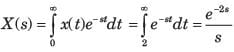QUESTION: 2

### Laplace transform of t2 sin⁡(2t).

Solution:

We know that,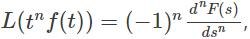Here, f(t) = sin⁡(2t) => F(s) =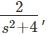Hence,  L(t2sin(2t)) =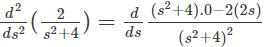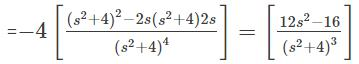QUESTION: 3

### Determine the Laplace transform of given signal. Q. x(t) = e-2tu(t+1)

Solution:

Laplace transform e-at u(t) = 1/s+a

for all Re(s) > - Re(a)

e-2t u(t+1) = 1/(s+2)

QUESTION: 4

Determine the Laplace transform of given signal.
Q. x(t) = e2tu(-t+2)

Solution: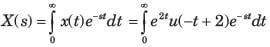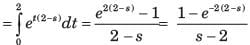QUESTION: 5

Determine the Laplace transform of given signal.
Q. x(t) = sin 5 t

Solution: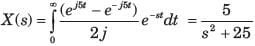QUESTION: 6

Determine the Laplace transform of given signal.
Q. x( t) = u(t) - u(t - 2)

Solution: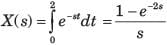QUESTION: 7

Determine the Laplace transform of given signal.
Q. x( t) = d/dt {te-1u(t)}

Solution: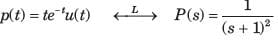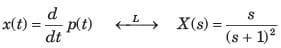QUESTION: 8

Determine the Laplace transform of given signal.
Q. x( t) = tu(t)*cos2πt u(t)

Solution: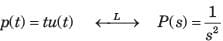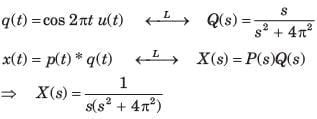QUESTION: 9

Determine the Laplace transform of given signal.
Q. x( t) = t3u(t)

Solution: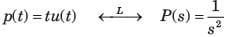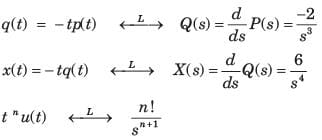QUESTION: 10

Determine the Laplace transform of given signal.
Q. x(t) = u( t - 1) * e-2tu( t - 1)

Solution:

L{−e-at u(t)}

= ∫(∞ to −∞) −e-at u(t)e-stdt

L{−e-at u(t)}

= ∫(∞ to 0) − e-(s+a)t dt

= - |e-(s+a)t/−(s+a)|∞ to 0

= −1/s+a

=> L{−e-2t u(t)} = -1/s+2

=> L{−e-2t u(t-1)} = e-(s+2)/s2 …………(1)

q(t) = L{u(t-1)}

= e-s/(s2)................(2)

Multiply (1) and (2)

= e-2(s+1)/(s+2)Use Code STAYHOME200 and get INR 200 additional OFF Use Coupon Code